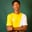Related Tags

python

# How to find a frequent element in a string with PythonShittu Olumide Ayodeji

### Overview

In Python, finding items frequently occurring in a string is challenging. However, Python offers a solution for it. Consider the scenario where we've got a list of strings and want to find out which words or letters frequently appear in each string. These frequent elements are never straightforward but can be found rapidly with Python.

We'll make use of the count(), max(), set(), and counter() methods in Python to find frequently occurring items in a string:

1. The built-in count() method in python counts the number of occurrences of any value passed to it.
2. In Python, the max() method returns the highest value inside an iterable or the highest of two or more inputs.
3. In Python, the set() method extends the concept of set. It is a mathematical word for a sequence of unique languages that may be easily created. The set() method turns iterable into a set of distinct iterable items, often known as a Set.
4. Aside from the max() and set() methods in Python, we can also use the counter() method to find out how often each character in a string appears.

### The Python count() method

One of the built-in string functions is the first way to find how many times an element appears in a string. The count() method counts how many items are in a collection. The method takes one input, either a character or a substring, and returns the number of times that character appears in the string returned by the function.

text = 'The energy is high!'
print(text.count('e'))

### Explanation

This method is incredibly easy to put into practice. We'll create a variable text and assign a string value to it in the code sample above. Then we'll add the count() method to the variable by including the character we wanted to count in the count() method, and the result will be printed on the console.

### The Python max() and set() methods

#let's create a variable with a string value
ab = 'Attitude'

#
most_frequent = max(set(ab), key = ab.count)
print('The most frequent letter is, ', most_frequent)



### Explanation

In the code widget above, we set the variable ab to a string value Attitude. To find the most frequent element in the string, the max() function takes in two attributes which are the set() method and the key.

Next, we'll pass the variable using the set() method which separates all the string alphabets while the variable key counts the number of times a specified value appears in the string.

The output is printed in the final line of the code.

### The Counter() class

from collections import Counter

text = "Hippopotamus"
print(text)

final = Counter(text)
final = max(final, key=final.get)

print("The most frequent letter is, ", final)


### Explanation

First, we've to import Counter() into our program. Since Counter() works with hashable objects, it requires a key to access these objects. We'll use the get() function to obtain the key.

The Counter() method helps determine the frequency of each character in the string, and we'll use the max() function to get the maximum count number.

### Using Python regular expressions

Finally, let's make use of regular expression (regex) to find how frequently an element appears in a Python string.

Note: This is an unusual approach when compared to other methods discussed above.

import re
text = 'Programming is fun'
print(len(re.findall('m', text)))

We'll utilize the regular expression module, notably the findall() method, to load the indices of where the character or substring occurs. Finally, we'll use the Python’s built-in len() method to look at the occurrence of the character or substring.

RELATED TAGS

python

CONTRIBUTORShittu Olumide Ayodeji
RELATED COURSES

View all Courses

Keep Exploring

Learn in-demand tech skills in half the time